문제1576--And Then There Was One

### 1576: And Then There Was One

실행시간 제한: 1 Sec  메모리사용 제한: 128 MB
제출: 25  통과: 18
[제출] [채점기록] [묻고답하기]

#### 문제 설명

Let's play a stone removing game.

Initially, n stones are arranged on a circle and numbered 1,..., n clockwise (Figure 1). You are also given two numbers k and m . From this state, remove stones one by one following the rules explained below, until only one remains. In step 1, remove stone m . In step 2, locate the k -th next stone clockwise from m and remove it. In subsequent steps, start from the slot of the stone removed in the last step, make k hops clockwise on the remaining stones and remove the one you reach. In other words, skip (k - 1) remaining stones clockwise and remove the next one. Repeat this until only one stone is left and answer its number.

For example, the answer for the case n = 8 , k = 5 , m = 3 is 1, as shown in Figure 1.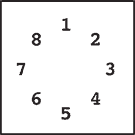Initial state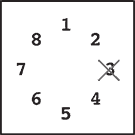Step 1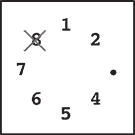Step 2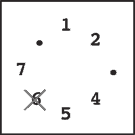Step 3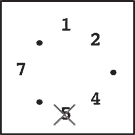Step 4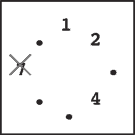Step 5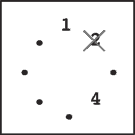Step 6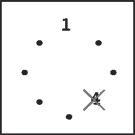Step 7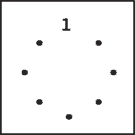Final state

Figure 1: An example game

Initial state: Eight stones are arranged on a circle.

Step 1: Stone 3 is removed since m = 3 .

Step 2: You start from the slot that was occupied by stone 3. You skip four stones 4, 5, 6 and 7 (since k = 5 ), and remove the next one, which is 8.

Step 3: You skip stones 1, 2, 4 and 5, and thus remove 6. Note that you only count stones that are still on the circle and ignore those already removed. Stone 3 is ignored in this case.

Steps 4-7: You continue until only one stone is left. Notice that in later steps when only a few stones remain, the same stone may be skipped multiple times. For example, stones 1 and 4 are skipped twice in step 7.

Final State: Finally, only one stone, 1, is on the circle. This is the final state, so the answer is 1.

#### 입력 설명

The input consists of multiple datasets each of which is formatted as follows.

n k m

The last dataset is followed by a line containing three zeros. Numbers in a line are separated by a single space. A dataset satisfies the following conditions.

2 <= n <= 10000, 1 <= k <= 10000, 1 <= m <= n

The number of datasets is less than 100.

#### 출력 설명

For each dataset, output a line containing the stone number left in the final state. No extra characters such as spaces should appear in the output.

#### 입력 예시 Copy

8 5 3
100 9999 98
10000 10000 10000
0 0 0


#### 출력 예시 Copy

1
93
2019